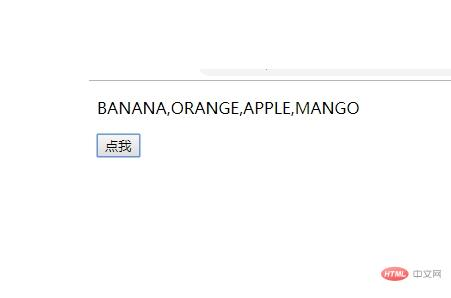# JavaScript 数组前端控 更新时间：2019-02-14 11:27:05

JavaScript Array（数组） 对象

```var car1="Saab";
var car2="Volvo";
var car3="BMW";```

1: 常规方式:

```var myCars=new Array();
myCars="Saab";
myCars="Volvo";
myCars="BMW";```

2: 简洁方式:

`var myCars=new Array("Saab","Volvo","BMW");`

3: 字面:

`var myCars=["Saab","Volvo","BMW"];`

`var name=myCars;`

`myCars="Opel";`

 是数组的第一个元素。 是数组的第二个元素。

```myArray=Date.now;
myArray=myFunction;
myArray=myCars;```

```var x=myCars.length // myCars 中元素的数量
var y=myCars.indexOf("Volvo")   // "Volvo" 值的索引值```

## 实例

```<script>
Array.prototype.myUcase=function(){
for (i=0;i<this.length;i++){
this[i]=this[i].toUpperCase();
}
}
function myFunction(){
var fruits = ["Banana", "Orange", "Apple", "Mango"];
fruits.myUcase();
var x=document.getElementById("demo");
x.innerHTML=fruits;
}
</script>```php中文网
phpstudy

QQ交流群